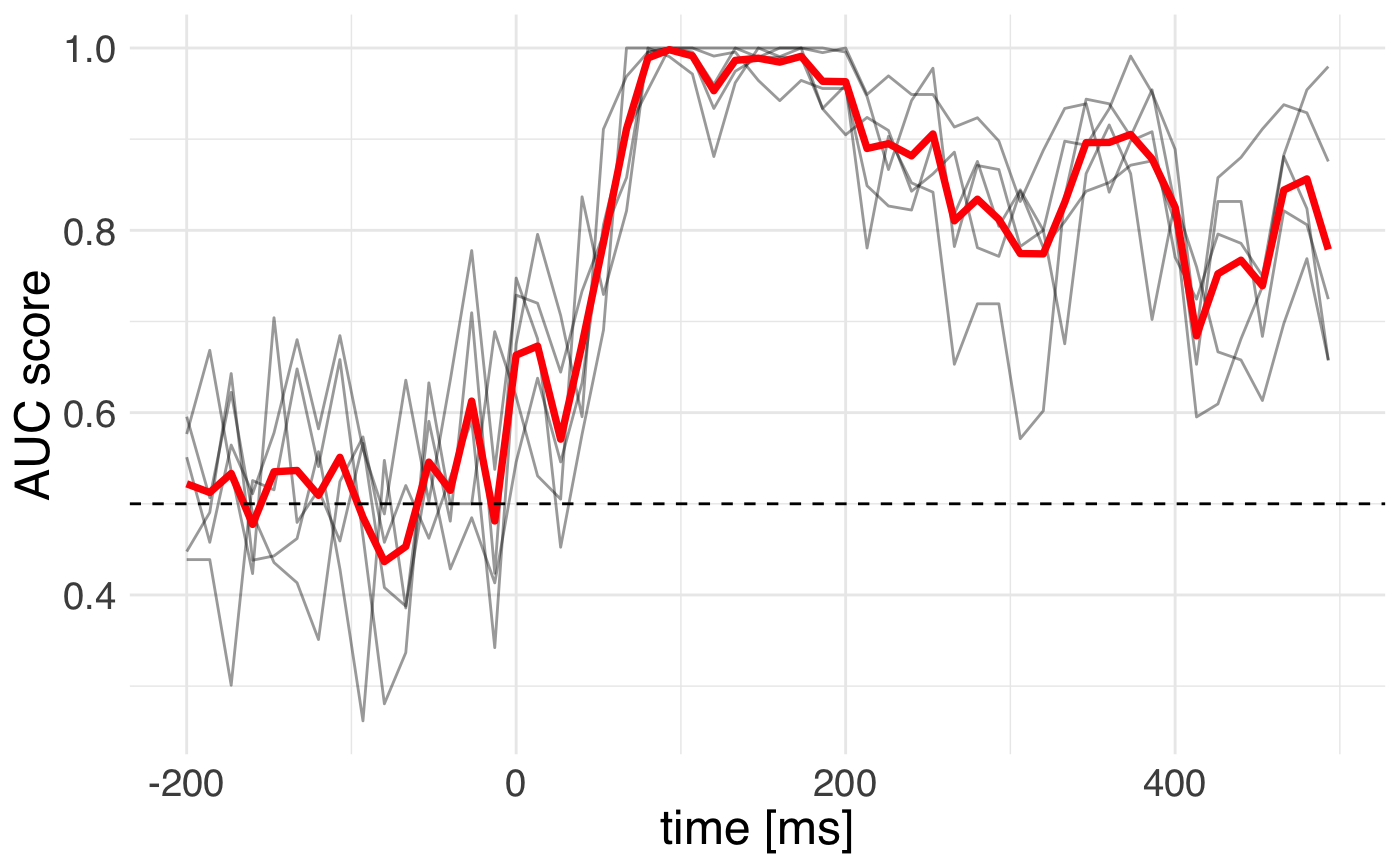In this example we are going to use the machine learning functionality of MNE-Python and scikit-learn to analyze evoked responses.

library(tidyverse)
library(mne)
## Importing MNE version=0.18.dev0, path='/Users/dengeman/github/mne-python/mne'

Let’s read in the raw data.

Let’s read in and preprocess the data.

## <Raw  |  sample_audvis_filt-0-40_raw.fif, n_channels x n_times : 376 x 41700 (277.7 sec), ~123.3 MB, data loaded>

We can set up the model. For this we will need to do a few imports from scikit-learn.

Time for action with R to plot the outputs!We can see that the cross-validation uncertainty is lowest around 150 milliseconds.